# 机器学习-吴恩达：学习笔记及总结（9）

## Coursera | Machine Learning | Andrew Ng

Thistledown    June 24, 2020

Course Link ：Week9 - Anomaly Detection & Recommender Systems

## 28 Density Estimation

#### 28.1 Problem Motivation

• Anomaly detection is a reasonably commonly used type of machine learning application

• Can be thought of as a solution to an unsupervised learning problem
• But, has aspects of supervised learning
• What is anomaly detection?

• Imagine you’re an aircraft engine manufacturer
• As engines roll off your assembly line you’re doing QA
• Measure some features from engines (e.g. heat generated and vibration)
• You now have a dataset of $x^1$ to $x^m$ (i.e. $m$ engines were tested)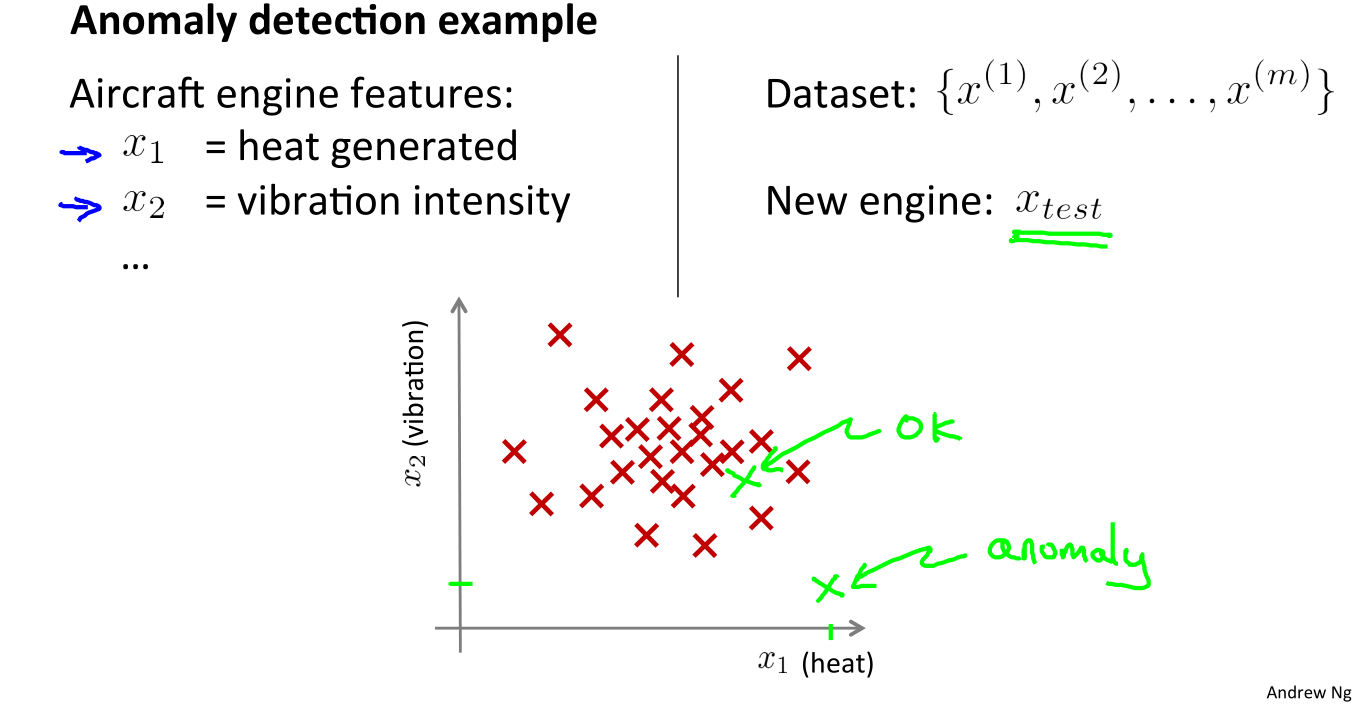• More formally

• We have a dataset which contains normal (data)
• How we ensure they’re normal is up to us
• In reality it’s OK if there are a few which aren’t actually normal
• Using that dataset as a reference point we can see if other examples are anomalous
• How do we do this?

• First, using our training dataset we build a model
• We can access this model using $p(x)$
• This asks, “What is the probability that example $x$ is normal”
• Having built a model
• if $p(x_{text}) < \varepsilon$, flag this as an anomaly
• if $p(x_{text}) \ge \varepsilon$, this is OK
• $\epsilon$ is some threshold probability value which we define, depending on how sure we need/want to be
• Applications

• Fraud detection
• $x^{(i)}$ = features of user $i$’s activities
• Model $p(x)$ from data
• Identify unusual user by checking which have $p(x) < \varepsilon$
• Manufacturing
• Monitoring computers in a data center
• $x^{(i)}$ = features of machine $i$

#### 28.2 Gaussian Distribution

• Also called the normal distribution
• $X \sim N(\mu, \sigma^2)$
• $p(x;\mu,\sigma) = \frac{1}{\sqrt{2\pi} \sigma} \exp\left( - \frac{(x - \mu)^2}{2\sigma^2} \right)$
• $\mu$: mean
• $\sigma^2$: variance
• $\sigma$: the standard deviation
• examples: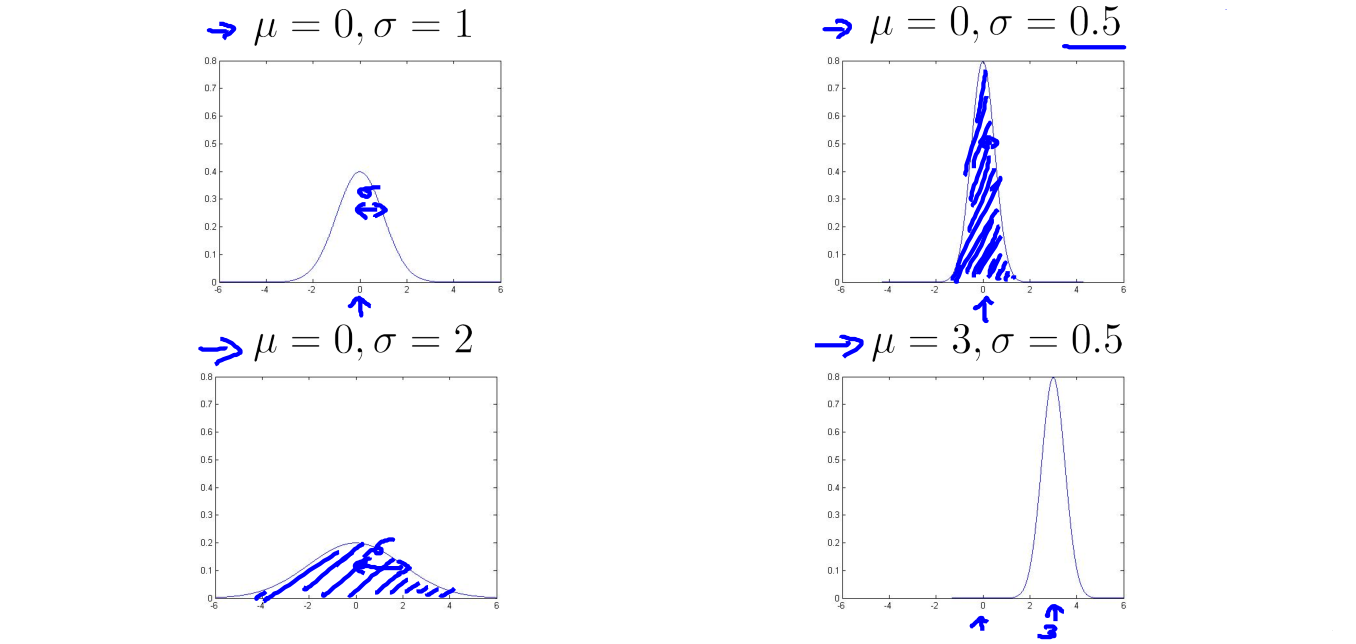• Parameter estimation
• Dataset: $\lbrace x^{(1)}, x^{(2)}, \dots, x^{(m)} \rbrace \quad x^{(i)}\in\Bbb{R}$
• $\mu = \frac{1}{m} \sum_{i=1}^m x^{(i)}$
• $\sigma^2 = \frac{1}{m} \sum_{i=1}^m \left( x^{(i)}-\mu \right)^2$
• or $\sigma^2 = \frac{1}{m-1} \sum_{i=1}^m \left( x^{(i)}-\mu \right)^2$

#### 28.3 Algorithm

• Density estimation

• Training set: $\lbrace x^{(1)}, \dots, x^{(m)}$, each example is $x \in \Bbb{R}^n$

• $x_1 \sim N(\mu_1, \sigma_1^2), \dots, x_n \sim N(\mu_n, \sigma_n^2)$

• So

• Anomaly detection algorithm

• Choose features $x_i$ that you think might be indicative of anomalous examples

• Fit parameters $\mu_1, \dots, \mu_n, \sigma_1^2, \dots, \sigma_n^2$

• Given new example $x$, compute $p(x)$:

• Anomaly if $p(x) < \varepsilon$

• Anomaly detection example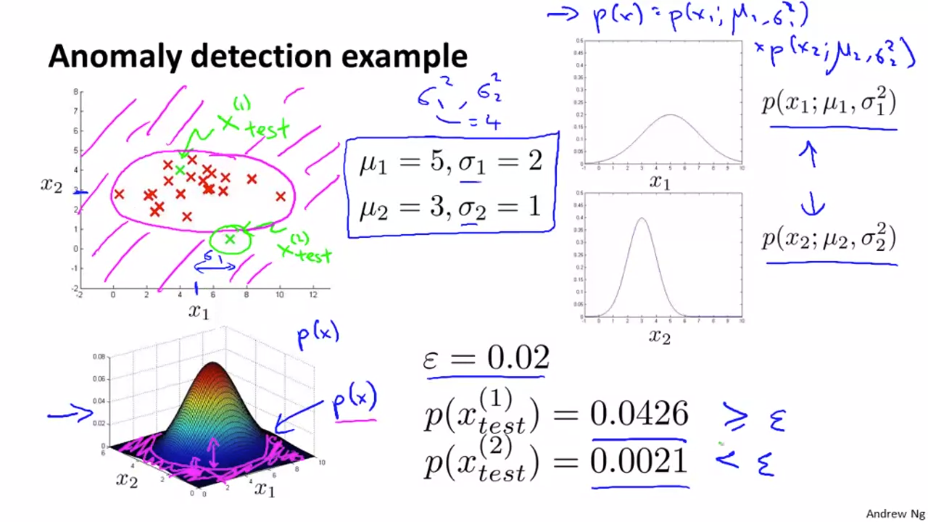## 29 Building an Anomaly Detection System

#### 29.1 Developing and Evaluating an Anomaly Detection System

• The importance of real-number evaluation

• When developing a learning algorithm (choosing features etc.), making decisions is much easier if we have a way of evaluating our learning algorithm
• Assume we have some labeled data, of anomalous and non-anomalous examples. ($y=0$ if normal, $y=1$ if anomalous)
• Training set: $x^{(1)}, x^{(2)}, \dots, x^{(m)}$ (assume normal examples/not anomalous)
• Cross validation set: $(x_{cv}^{(1)}, y_{cv}^{(1)}), \dots, (x_{cv}^{(m_{cv})}, y_{cv}^{(m_{cv})})$
• Test set: $(x_{test}^{(1)}, y_{test}^{(1)}), \dots, (x_{test}^{(m_{test})}, y_{test}^{(m_{test})})$
• Algorithm evaluation

• Fit model $p(x)$ on training set $\lbrace x^{(1)}, \dots, x^{(m)} \rbrace$

• On a cross validation/test example $x$, predict

• Possible evaluation metrics:

• True positive, false positive, false negative, true negative
• Precision/Recall
• $F_1$-score
• Can also use cross validation set to choose parameter $\varepsilon$

#### 29.2 Anomaly Detection vs. Supervised Learning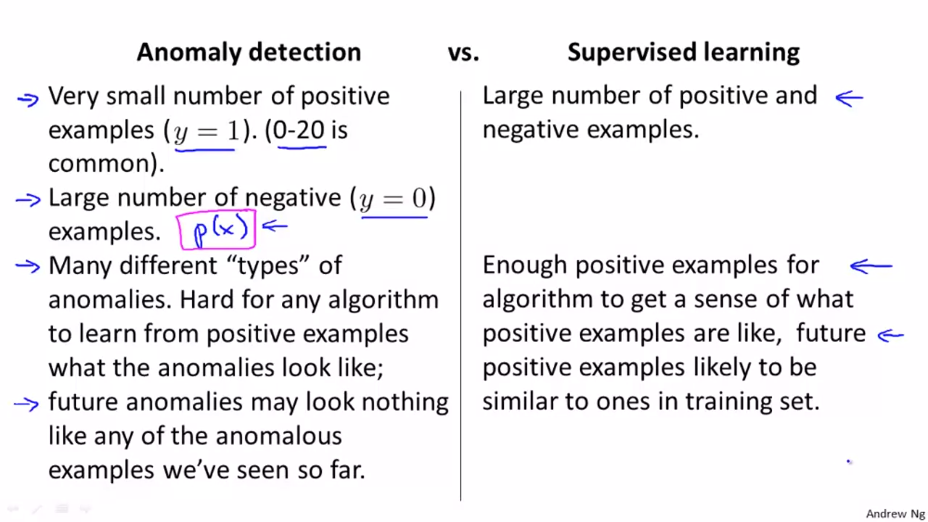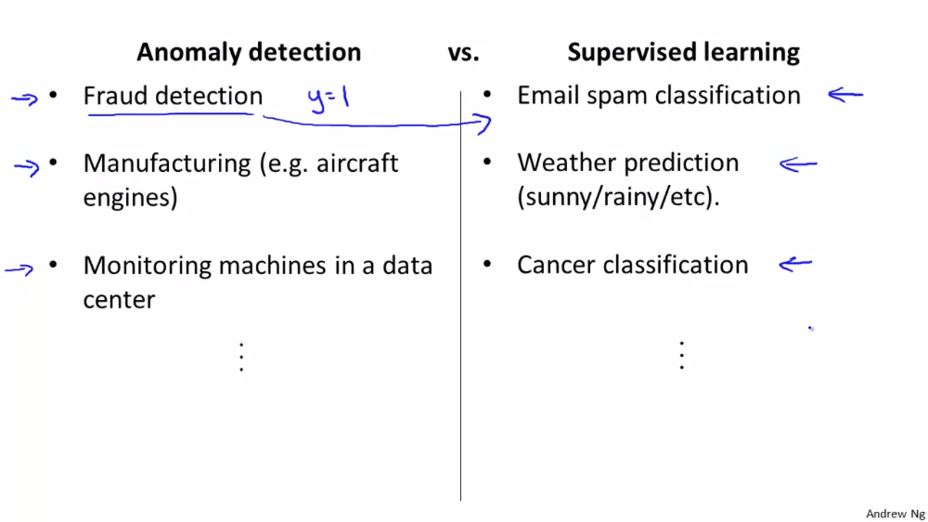#### 29.3 Choosing What Features to Use

• Non-Gaussian features

• Non-Gaussian data might look like this: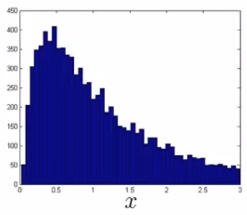• Can play with different transformations of the data to make it look more Gaussian:

• $x \leftarrow \log(x + c)$
• $x \leftarrow x^{\text{small number}}$
• Error analysis for anomaly detection

• Want:
• $p(x)$ large for normal examples $x$
• $p(x)$ small for anomalous examples $x$
• Most common problems:
• $p(x)$ is comparable (say, both large) for normal and anomalous examples
• Solve (like supervised learning error analysis procedure):
• Run algorithm on cross validation set
• See which one it got wrong
• Try coming up with more features to distinguish between the normal and the anomalous examples

## 30 Multivariate Gaussian Distribution

#### 30.1 Multivariate Gaussian Distribution

• Multivariate Gaussian (Normal) distribution

• $x \in \Bbb{R}^n$. Don’t model $p(x_1), p(x_2), \dots$, etc. separately.

• Model $p(x)$ all in one go

• Parameters: $\mu \in \Bbb{R}^n$, $\Sigma \in \Bbb{R}^{n \times n}$ (covariance matrix)

• Formula:

• Multivariate Gaussian (Normal) examples: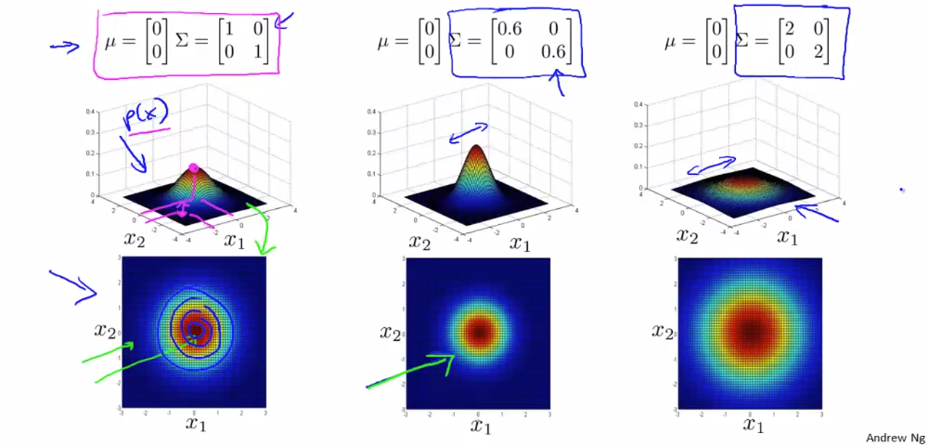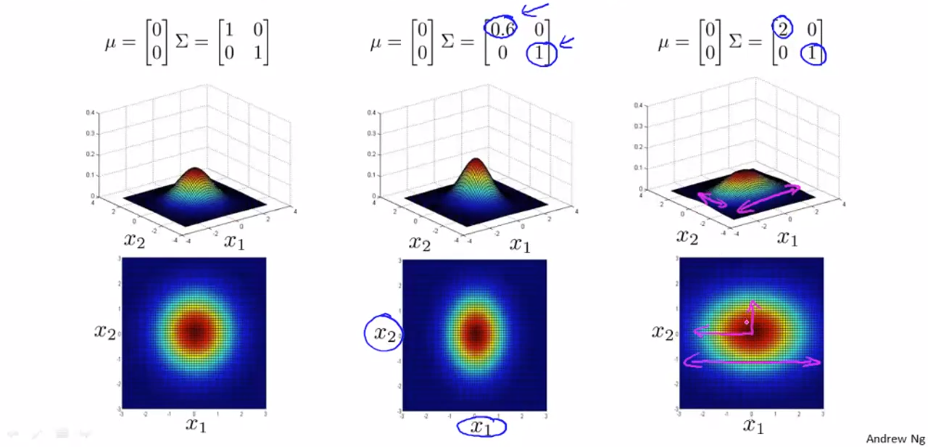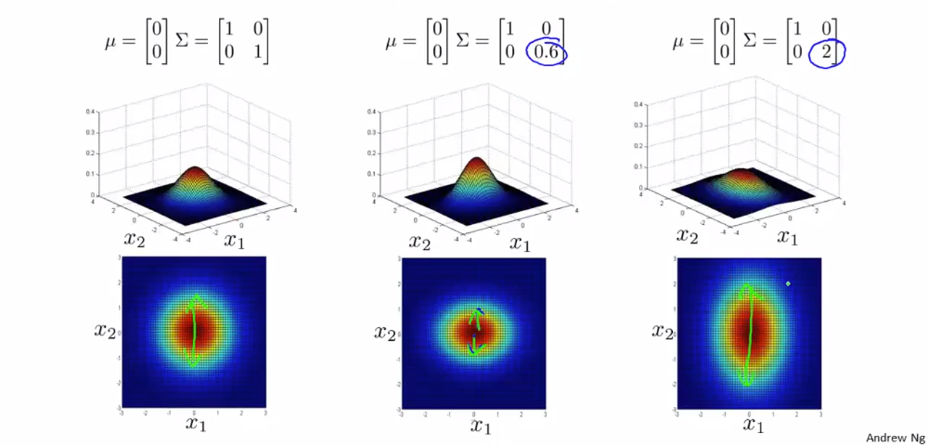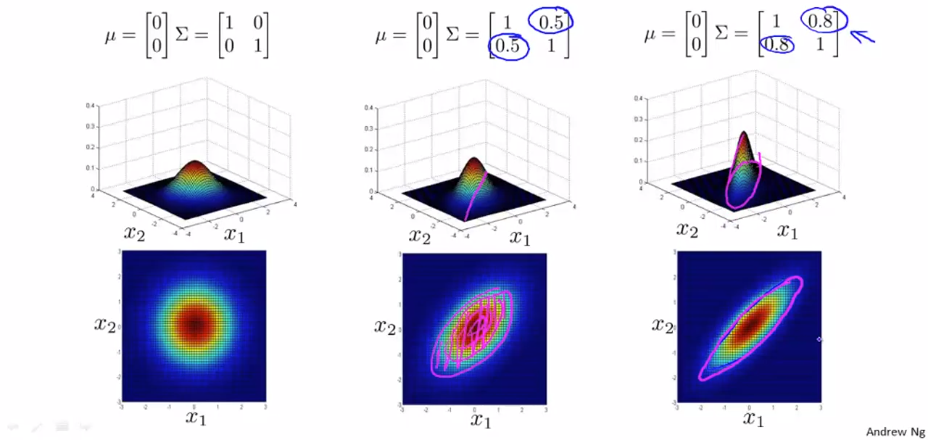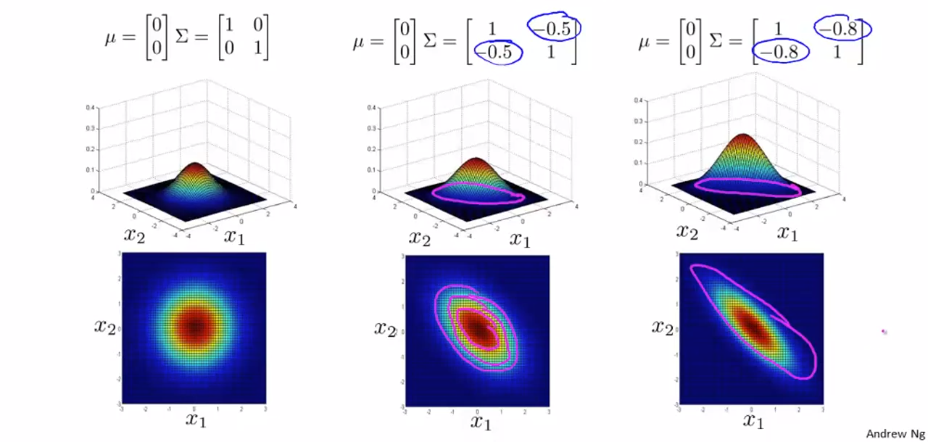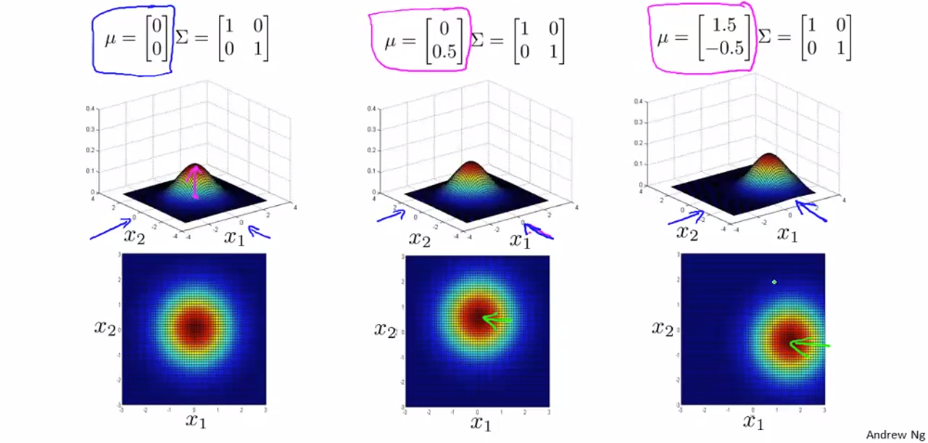#### 30.2 Anomaly Detection using the Multivariate Gaussian Distribution

• Multivariate Gaussian (Normal) distribution

• Formula:

• Parameters $\mu, \Sigma$
• Given training set $\lbrace x^{(1)}, x^{(2)}, \dots, x^{(m)} \rbrace$

• Parameter fitting

• Anomaly detection with the multivariate Gaussian

• Fit model $p(x)$ by setting

• Given a new example $x$, compute

• Flag an anomaly of $p(x) < \varepsilon$

• Relationship to original model

• Original model: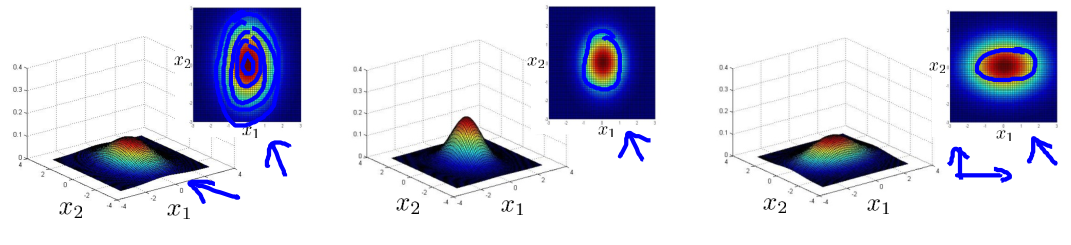• Corresponds to multivariate Gaussian:

• Where $% $
• Comparison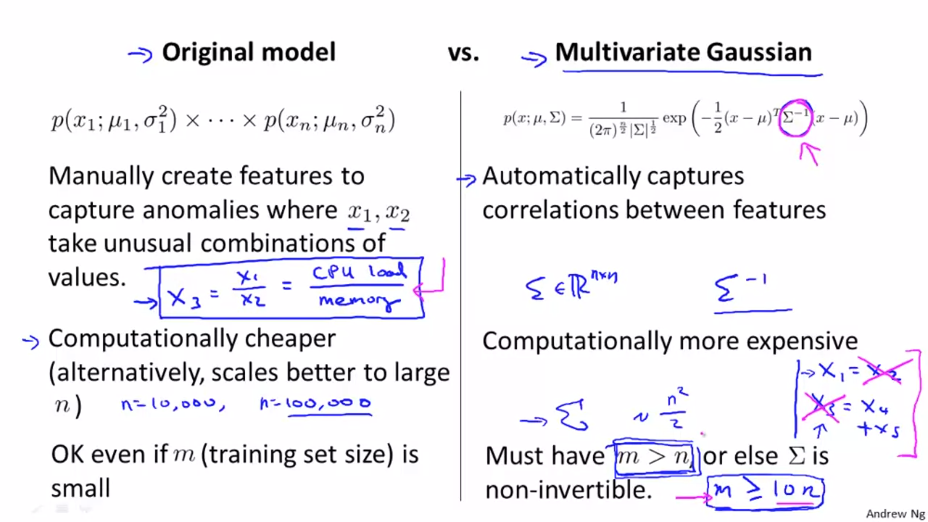## 31 Predicting Movie Ratings

#### 31.1 Problem Formulation

• Example: Predicting movie ratings

• User rates movies using zero to five stars• And we have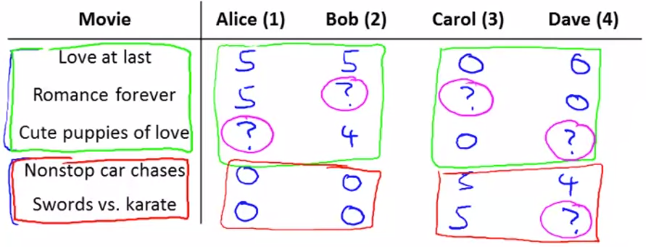• Let

• $n_u$ = no. users
• $n_m$ = no. movies
• $r(i, j) = 1$ if user $j$ has rated movie $i$
• $y(i, j)$ = rating given by user $j$ to movie $i$ (defined only if $r(i,j)=1$)
• The problem is as follows

• Given $r(i,j)$ and $y(i,j)$, go through and try and predict missing values
• Come up with a learning algorithm that can fill in these missing values

#### 31.2 Content Based Recommendations

• Using our example above, how do we predict?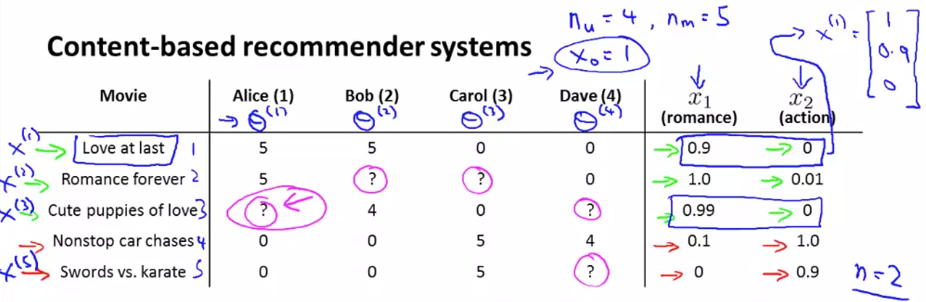• For each movie we have a feature which measure degree to which each film is a
• Romance ($x_1$)
• Action ($x_2$)
• If we have features like these, each film can be recommended by a feature vector
• Add an extra feature which is $x_0 = 1$ for each film
• So for each film we have a $[3 \times 1]$ vector, which for film number 1 (“Love at Last”) would be $x^{(1)} = \begin{bmatrix} 1 & 0.9 & 0 \end{bmatrix}^T$
• i.e. for our dataset we have $\lbrace x^{(1)}, x^{(2)}, x^{(3)}, x^{(4)}, x^{(5)} \rbrace$
• Where each of these is a $[3\times 1]$ vector with an $x_0 = 1$ and then a romance and an action score
• We could treat each rating for each user as a separate linear regression problem
• For each user $j$ we could learn a parameter vector $\theta^{(j)} \in \Bbb{R}^{n+1}$
• Then predict that user $j$ will rate movie $i$ with $(θ^{(j)})^T x^{(i)} = \text{stars}$
• All we’re doing here is applying a linear regression method for each user
• So we determine a future rating based on their interest in romance and action based on previous films
• We should also add one final piece of notation
• $m^{(j)}$, number of movies rated by the user $j$
• Problem formulation

• Notations:

• $r(i, j) = 1$ if user $j$ has rated movie $i$
• $y(i, j)$ = rating given by user $j$ to movie $i$ (if defined)
• $\theta^{(j)} \in \Bbb{R}^{n+1}$ = parameters vector for user $j$
• $x^{(i)}\in \Bbb{R}^{n+1}$ = feature vector for movie $i$
• $m^{(j)}$ = no. of movies rated by user $j$
• For user $j$, movie $i$, predict rating: $\left( \theta^{(j)} \right)^T x^{(i)}$

• To learn $\theta^{(j)}$:
$\min_{\theta^{(j)}} \frac{1}{2m^{(j)}} \sum_{i:r(i, j)=1}\left[\left( \theta^{(j)} \right)^T x^{(i)} - y^{(i, j)} \right]^2 + \frac{\lambda}{2 m^{(j)}} \sum_{k=1}^n \left( \theta_k^{(j)} \right)^2$

• $m^{(j)}$ is a constant value - you can remove it
• Optimization objective

• To learn $\theta^{(j)}$ (parameter for user $j$):

• To learn $\theta^{(1)}, \theta^{(2)}, \dots, \theta^{(n_u)}$

• Optimization algorithm

## 32 Collaborative Filtering

#### 32.1 Collaborative Filtering

• The collaborative filtering algorithm has a very interesting property - does feature learning

• i.e. it can learn for itself what features it needs to learn
• Recall our original data set above for our five films and four raters

• Here we assume someone had calculated the “romance” and “action” amounts of the films
• This can be very hard to do in reality
• Often want more features than just two
• So - let’s change the problem and pretend we have a data set where we don’t know any of the features associated with the films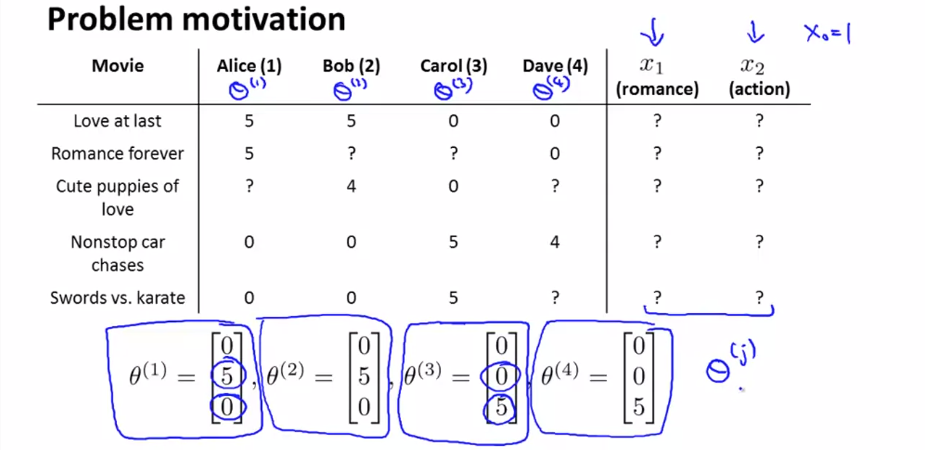• Now let’s make a different assumption
• We’ve polled each user and found out how much each user likes
• Romantic films
• Action films
• Which has generated the following parameter set
• Alice and Bob like romance but hate action
• Carol and Dave like action but hate romance
• If we can get these parameters from the users we can infer the missing values from our table

• Lets look at “Love at Last”
• Alice and Bob loved it
• Carol and Dave hated it
• We know from the feature vectors Alice and Bob love romantic films, while Carol and Dave hate them
• Based on the factor Alice and Bob liked “Love at Last” and Carol and Dave hated it we may be able to (correctly) conclude that “Love at Last” is a romantic film
• This is a bit of a simplification in terms of the math, but what we’re really asking is

• What feature vector $x^{(1)}$ should be - so that
• $(\theta^{(1)})^T x_1$ is about 5
• $(\theta^{(2)})^T x_1$ is about 5
• $(\theta^{(3)})^T x_1$ is about 0
• $(\theta^{(4)})^T x_1$ is about 0
• From this we can guess that $x^{(1)}$ may be $\begin{bmatrix} 1 & 1.0 & 0.0 \end{bmatrix}^T$
• Using that same approach we should then be able to determine the remaining feature vectors for the other films
• Formalizing the collaborative filtering problem

• Given $\theta^{(1)}, \dots, \theta^{(n_u)}$ (i.e. given the parameter vectors for each users’ preferences), to learn $x^{(i)}$

• We must minimize an optimization function which tries to identify the best parameter vector associated with a film

• So we’re summing over all the indices $j$ for where we have data for movie $i$
• We’re minimizing this squared error
• Like before, the above equation gives us a way to learn the features for one film

• We want to learn all the features for all the films - so we need an additional summation term
• How does this work with the previous recommendation system

• Content based recommendation systems
• Saw that if we have a set of features for movie rating you can learn a user’s preferences
• Now
• If you have your users preferences you can therefore determine a film’s features
• This is a bit of a chicken & egg problem
• What you can do is
• Randomly guess values for $\theta$
• Then use collaborative filtering to generate $x$
• Then use content based recommendation to improve $\theta$
• Use that to improve $x$
• And so on
• This actually works
• Causes your algorithm to converge on a reasonable set of parameters
• This is collaborative filtering
• We call it collaborative filtering because in this example the users are collaborating together to help the algorithm learn better features and help the system and the other users

#### 32.2 Collaborative Filtering Algorithm

• Here we combine the ideas from before to build a collaborative filtering algorithm

• Collaborative filtering optimization objective

• If we’re given the film’s features we can use that to work out the users’ preference:

• If we’re given the users’ preferences we can use them to work out the film’s features

• One thing you could do is

• Randomly initialize parameter
• Go back and forward

• But there’s a more efficient algorithm which can solve $\theta$ and $x$ simultaneously

• Define a new optimization objective which is a function of $x$ and $\theta$
• Goal:

• Understanding this optimization objective

• The squared error term is the same as the squared error term in the two individual objectives above $\sum_{(i, j):r(i, j)=1} \left[\left( \theta^{(j)} \right)^T x^{(i)} - y^{(i, j)} \right]^2$

• So it’s summing over every movie rated by every users
• Note the “$:$” means, “for which”
• Sum over all pairs $(i,j)$ for which $r(i,j)$ is equal to 1
• The regularization terms

• Are simply added to the end from the original two optimization functions
• This newly defined function has the property that

• If you held $x$ constant and only solved $\theta$ then you solve the, “Given $x$, solve $\theta$” objective above
• Similarly, if you held $\theta$ constant you could solve $x$
• In order to come up with just one optimization function we treat this function as a function of both film features $x$ and user parameters $\theta$

• Only difference between this in the back-and-forward approach is that we minimize with respect to both $x$ and $\theta$ simultaneously
• When we’re learning the features this way

• Previously had a convention that we have an $x_0 = 1$ term

• When we’re using this kind of approach we have no

$x_0$,

• So now our vectors (both $x$ and $\theta$) are $n$-dimensional (NOT $n+1$)
• i.e. $x \in \Bbb{R}^n, \theta \in \Bbb{R}^n$
• We do this because we are now learning all the features so if the system needs a feature always = 1 then the algorithm can learn one

• Collaborative filtering algorithm

• 1) Initialize $\theta^{(1)}, \dots, \theta^{(n_u)}$ and $x^{(1)}, \dots, x^{(n_m)}$ to small random values

• A bit like neural networks - initialize all parameters to small random numbers
• 2) Minimize cost function $J(x^{(1)}, \dots, x^{(n_m)},\theta^{(1)}, \dots, \theta^{(n_u)})$ using gradient descent

• 3) Having minimized the values, given a user (user $j$) with parameters $\theta$ and movie (movie $i$) with learned features $x$, we predict a star rating of $\theta^Tx$

## 33 Low Rank Matrix Factorization

#### 33.1 Vectorization: Low Rank Matrix Factorization

• low rank matrix factorization
• $Y = \begin{bmatrix} \left(\theta^{(1)}\right)^{T}\left(x^{(1)}\right) & \left(\theta^{(2)}\right)^{T}\left(x^{(1)}\right) & \dots & \left(\theta^{\left(n_{u}\right)}\right)^{T}\left(x^{(1)}\right) \newline \left(\theta^{(1)}\right)^{T}\left(x^{(2)}\right) & \left(\theta^{(2)}\right)^{T}\left(x^{(2)}\right) & \dots & \left(\theta^{\left(n_{u}\right)}\right)^{T}\left(x^{(2)}\right) \newline \vdots & \vdots & \ddots & \vdots \newline \left(\theta^{(1)}\right)^{T}\left(x^{\left(n_{m}\right)}\right) & \left(\theta^{(2)}\right)^{T}\left(x^{\left(n_{m}\right)}\right) & \dots & \left(\theta^{\left(n_{u}\right)}\right)^{T}\left(x^{\left(n_{m}\right)}\right) \end{bmatrix}$
• $X = \begin{bmatrix}-\left( x^{(1)} \right)^T- \\ \cdots \\ -\left( x^{(n_m)} \right)^T- \end{bmatrix}, \Theta = \begin{bmatrix}-\left( \theta^{(1)} \right)^T- \\ \cdots \\ -\left( \theta^{(n_u)} \right)^T- \end{bmatrix}$
• $Y = X\Theta^T$
• Find related movies
• For each product $i$, we learn a feature vector $x^{(i)} \in \Bbb{R}^n$
• How to find movie $j$ related to movie $i$ ?
• $\text{small } |x^{(i)} - x^{(j)}| \rightarrow \text{movie$j$and$i$are “similar”}$

#### 33.2 Implementational Detail: Mean Normalization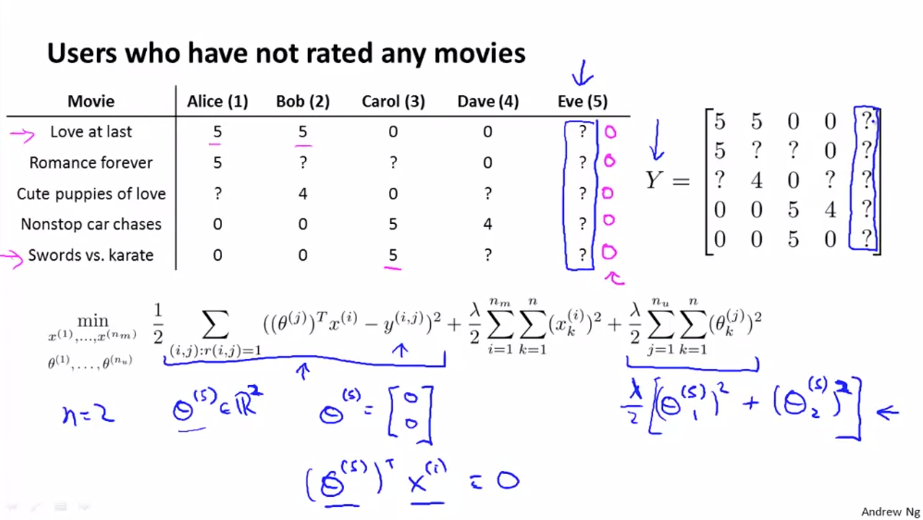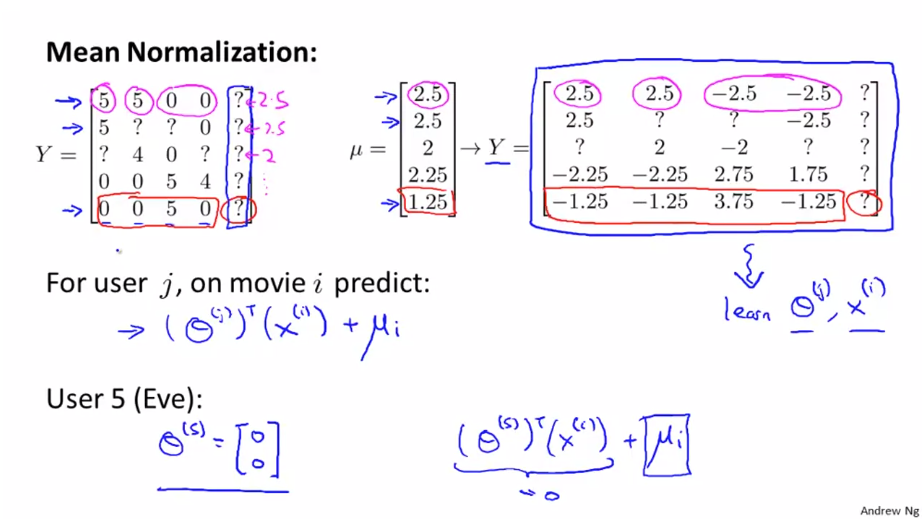## Ex8: Anomaly Detection and Recommender System👨‍💻

See this exercise on Coursera-MachineLearning-Python/ex8/ex8.ipynb

Thistledown